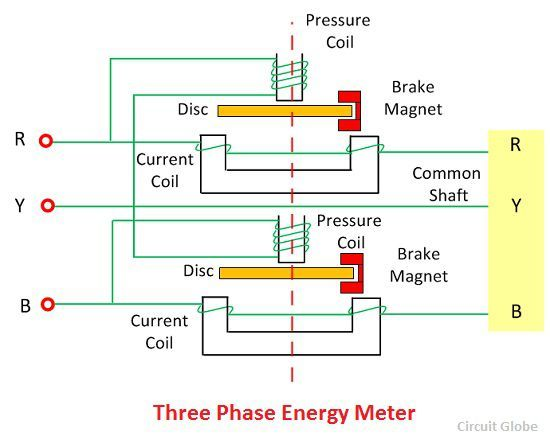# Three Phase Energy Meter

Definition: The meter which is used for measuring the power of three phase supply is known as the three phase energy meter. The three phase meter is constructed by connecting the two single phase meter through the shaft. The total energy is the sum of the reading of both the elements.

## Working Principle of Three Phase Energy Meter

The torque of both the elements is added mechanically, and the total rotation of the shaft is proportional to the three phase energy consumption.

## Construction of Three Phase Energy Meter

The three phase energy meter has two discs mounted on the common shaft. Both the disc has its braking magnet, copper ring, shading band and the compensator for getting the correct reading. The two elements are used for measuring the three phase power. The construction of the three phase meter is shown in the figure below.For three phase meter, the driving torque of both the elements is equal. This can be done by adjusting the torque. The torque is adjusted by connecting the current coils of both the elements in the series and their potential coils in parallel. The full load current is passed through the coil due to which the two opposite torque is set up in the coil.

The strength of both the torques are equal, and hence they do not allow the disc to rotate. If the torque becomes unequal and the disc rotates then the magnetic shunt is adjusted. The balance torque is obtained before testing the meter. The position of the compensator and the braking magnet are separately adjusted to each of the element for obtaining the balance torque.

### 4 thoughts on “Three Phase Energy Meter”

1. In the three phase energy meter ,in case of unbalanced load how the rotation of disc in the energy meter is made to run to calculate correct consumption of power

2. this is the in-depth information and working mechanism of this device. Nice site, subscribed for more upcoming posts.